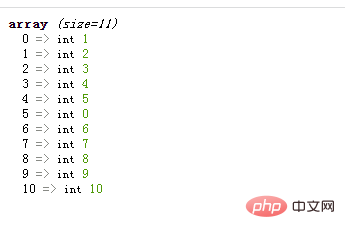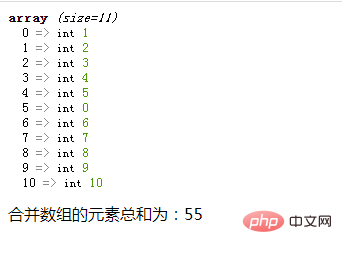# php怎么实现两个数组合并并且求和php实现两个数组合并并且求和可以看成两个部分：

• php实现两个数组合并

• php实现合并数组的求和

array_merge() 函数用于把一个或多个数组合并为一个数组，会返回一个包含两个数组元素的合并数组

```<?php
\$a1=array(1,2,3,4,5,0);
\$a2=array(6,7,8,9,10);
\$arr=array_merge(\$a1,\$a2);
var_dump(\$arr);
?>```array_sum(\$arr) 函数可以计算数组\$arr中所有元素的和

```<?php
\$a1=array(1,2,3,4,5,0);
\$a2=array(6,7,8,9,10);
\$arr=array_merge(\$a1,\$a2);
var_dump(\$arr);
\$sum=array_sum(\$arr);
echo "合并数组的元素总和为：".\$sum;
?>````array_sum()` 函数计算数组中所有元素的和

• 如果数组 arr 的所有元素都是整数，则返回一个整数值；如果其中有一个或多个值是浮点数，则返回浮点数。

• 如果数组 arr 中存在非数值类型的元素，那么 PHP 会尝试将它们转换成一个数值，转换失败就作为 0 值。例如，字符串 "45" 会被转换成整数 45，字符串 "12.4abc" 会被转换成小数 12.4。1/1